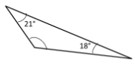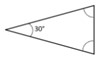# Finding an angle measure of a triangle given two angles

The sum of angles of a triangle is 180°.

In a triangle, we know there are three angles and three sides. In this lesson we solve for missing angle measure given two angle measures in a triangle.

Find the missing angle in the given triangle below. Sum of angles in a triangle is 180°.### Solution

Step 1:

Sum of angles in a triangle is 180°.

29° + 33° + b = 180°; 62° + b = 180°

Step 2:

b = 180° − 62° = 118°

So missing angle = 118°

Find the missing angle in the given triangle below. Sum of angles in a triangle is 180°.### Solution

Step 1:

Sum of angles in a triangle is 180°.

29° + 33° + b = 180°; 62° + b = 180°

Step 2:

b = 180° − 62° = 118°

So missing angle = 118°